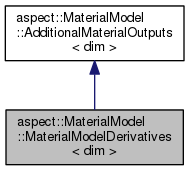ASPECT
aspect::MaterialModel::MaterialModelDerivatives< dim > Class Template Reference
Inheritance diagram for aspect::MaterialModel::MaterialModelDerivatives< dim >:[legend]

## Public Member Functions

MaterialModelDerivatives (const unsigned int n_points)Public Member Functions inherited from aspect::MaterialModel::AdditionalMaterialOutputs< dim >

virtual void average (const MaterialAveraging::AveragingOperation, const FullMatrix< double > &, const FullMatrix< double > &)

## Public Attributes

std::vector< double > viscosity_derivative_wrt_pressure

std::vector< SymmetricTensor< 2, dim > > viscosity_derivative_wrt_strain_rate

## Detailed Description

### template<int dim> class aspect::MaterialModel::MaterialModelDerivatives< dim >

This class holds the derivatives for the Newton solver.

Definition at line 42 of file newton.h.

## § MaterialModelDerivatives()

template<int dim>
 aspect::MaterialModel::MaterialModelDerivatives< dim >::MaterialModelDerivatives ( const unsigned int n_points )

Constructor. Initialize the various arrays of this structure with the given number of quadrature points.

## § viscosity_derivative_wrt_pressure

template<int dim>
 std::vector aspect::MaterialModel::MaterialModelDerivatives< dim >::viscosity_derivative_wrt_pressure

The derivatives of the viscosities

Definition at line 54 of file newton.h.

## § viscosity_derivative_wrt_strain_rate

template<int dim>
 std::vector > aspect::MaterialModel::MaterialModelDerivatives< dim >::viscosity_derivative_wrt_strain_rate

Definition at line 55 of file newton.h.

The documentation for this class was generated from the following file:
• /home/bob/source/include/aspect/newton.h